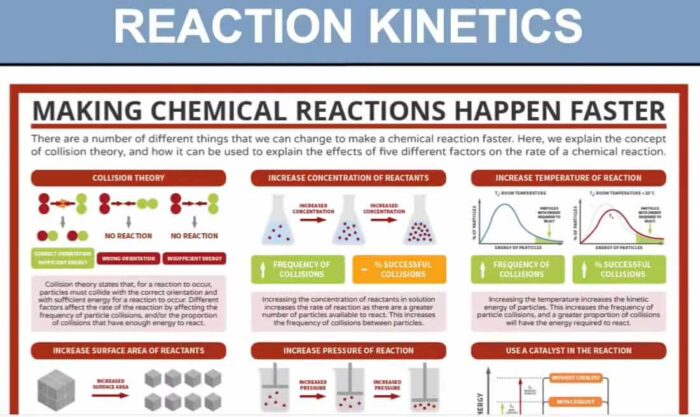Chemical kinetics, also known as reaction kinetics, is the branch of physical chemistry that is concerned with understanding the rates of chemical reactions. It is to be contrasted with chemical thermodynamics, which deals with the direction in which a reaction occurs but in itself tells nothing about its rate. Chemical kinetics includes investigations of how experimental conditions influence the speed of a chemical reaction and yield information about the reaction’s mechanism and transition states, as well as the construction of mathematical models that also can describe the characteristics of a chemical reaction.

## Course Contents

• Simple rate equations; orders of reaction; rate constants
• Concept of activation energy
• Effect of concentration, temperature, and catalysts on reaction rate
• Homogeneous and heterogeneous catalysis
• Enzymes as biological catalysts

## Learning Outcomes

Candidates should be able to:

• (a) explain and use the terms: rate of reaction; rate equation; order of reaction; rate constant; half-life of a reaction; rate-determining step; activation energy; catalysis
• (b) construct and use rate equations of the form rate = k[A]m[B]n (limited to simple cases of single-step reactions and of multi-step processes with a rate-determining step, for which m and n are 0, 1 or 2), including:
• (i) deducing the order of a reaction by the initial rates method
• (ii) justifying, for zero- and first-order reactions, the order of reaction from concentration-time graphs
• (iii) verifying that a suggested reaction mechanism is consistent with the observed kinetics
• (iv) predicting the order that would result from a given reaction mechanism
• (v) calculating an initial rate using concentration data [integrated forms of rate equations are not required]
• (c)
• (i) show understanding that the half-life of a first-order reaction is independent of concentration
• (ii) use the half-life of a first-order reaction in calculations
• (d) calculate a rate constant using the initial rates method
• (e) devise a suitable experimental technique for studying the rate of a reaction, from given information
• (f) explain qualitatively, in terms of frequency of collisions, the effect of concentration changes on the rate of a reaction
• (g) show understanding, including reference to the Boltzmann distribution, of what is meant by the term activation energy
• (h) explain qualitatively, in terms both of the Boltzmann distribution and of collision frequency, the effect of temperature change on a rate constant (and hence, on the rate) of a reaction
• (i)
• (i) explain that, in the presence of a catalyst, a reaction has a different mechanism, i.e. one of lower activation energy, giving a larger rate constant
• (ii) interpret this catalytic effect on a rate constant in terms of the Boltzmann distribution
• (j) outline the different modes of action of homogeneous and heterogeneous catalysis, including:
• (i) the Haber process
• (ii) the catalytic removal of oxides of nitrogen in the exhaust gases from car engines (see also Section 11.3)
• (iii) the catalytic role of atmospheric oxides of nitrogen in the oxidation of atmospheric sulfur dioxide
• (iv) catalytic role of Fe2+ in the I–/S2O82– reaction
• (k) describe enzymes as biological catalysts which may have specific activity
• (l) explain the relationship between substrate concentration and the rate of an enzyme-catalysed reaction in biochemical systems